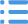# 四年级数学运算定律教案

作为一位杰出的教职工，编写教案是必不可少的，教案是备课向课堂教学转化的关节点。那么教案应该怎么写才合适呢？下面是小编精心整理的四年级数学运算定律教案，仅供参考，希望能够帮助到大家。一、教学内容：加法交换律和结合律P17――P18

二、教学目标：

1、在解决实际问题的过程中，发现并掌握加法交换律和结合律，学会用字母表示加法交换律和结合律。

2、在探索运算律的过程中，发展分析、比较、抽象、概括能力，培养学生的符号感。

3、培养学生的观察能力和概括能力。

三、教学重难点

重点：发现并掌握加法交换律、结合律。

难点：由具体上升到抽象，概括出加法交换律和加法结合律。

四、教学准备

多媒体课件

五、教学过程

（一）导入新授

1、出示教材第17页情境图。

师：在我们班里，有多少同学会骑自行车？你最远骑到什么地方？

师生交流后，课件出示李叔叔骑车旅行的场景：骑车是一项有益健康的运动，你看，这位李叔叔正在骑车旅行呢！

2、获取信息。

师：从中你知道了哪些数学信息？（学生回答）

3、师小结信息，引出课题：加法交换律和结合律。

（二）探索发现

第一环节探索加法交换律

1、课件继续出示：“李叔叔今天上午骑了40km，下午骑了56km，一共骑了多少千米？”

学生口头列式，教师板书出示：40+56=96（千米）56+40=96（千米）

你能用等号把这两道算式写成一个等式吗？40+56=56+40

你还能再写出几个这样的等式吗？

学生独自写出几个这样的等式，并在小组内交流各自写出的等式，互相检验写出的等式是否符合要求。

2、观察写出的这些算式，你有什么发现？并用自己喜欢的方式表示出来。

全班交流。从这些算式可以发现：两个数相加，交换加数的位置，和不变。

可以用符号来表示：△+☆=☆+△；

可以用文字来表示：甲数十乙数=乙数十甲数。

3、如果用字母a、b分别表示两个加数，又可以怎样来表示发现的这个规律呢？

a+b=b+a

教师指出：这就是加法交换律。

4、初步应用：在（）里填上合适的数。

37+36=36+（）305+49=（）+305b+100=（）+b

47+（）=126+（）m+（）=n+（）13+24=（）+（）第二环节探索加法结合律

1、课件出示教材第18页例2情境图。

师：从例2的情境图中，你获得了哪些信息？

师生交流后提出问题：要求“李叔叔三天一共骑了多少千米”可以怎样列式？

学生独立列式，指名汇报。

汇报预设：

方法一：先算出“第一天和第二天共骑了多少千米”：

（88+104）+96

=192+96

=288（千米）

方法二：先算出“第二天和第三天共骑了多少千米”：

88+（104+96）

=88+200

=288（千米）

把这两道算式写成一道等式：

（88+104）+96=88+（104+96）

2、算一算，下面的.○里能填上等号吗？

（45+25）+13○45+（25+13）（36+18）+22○36+（18+22）

小组讨论。先比较每组的两个算式，再比较这三组算式，在小组里说说你有什么发现。

集体交流，使学生明确：三个算式加数没变，加数的位置也没变，运算的顺序变了，它们的和不变。也就是：三个数相加，先把前两个数相加，或者先把后两个数相加，和不变。

3、如果用字母a、b、c分别表示三个加数，可以怎样用字母来表示这个规律呢？

（a+b）+c=a+（b+c）

教师指出：这就是加法结合律。

4、初步应用。

在横线上填上合适的数。

（45+36）+64=45+（36+）

（560+）+=560+（140+70）

（360+）+108=360+（92+）

（57+c）+d=57+（+）

（三）巩固发散

1、完成教材第18页“做一做”。

学生独立填写，组织汇报时，让学生说说是根据什么运算律填写的。

2、下面各等式哪些符合加法交换律，哪些符合加法结合律？

（1）470+320=320+470

（2）a+55+45=55+45+a

（3）（27+65）+35=27+（65+35）

（4）70+80+40=70+40+80

（5）60+（a+50）=（60+a）+50

（6）b+900=900+b

（四）评价反馈

通过今天这节课的学习，你有哪些收获？

师生交流后总结：学习了加法交换律和结合律，并知道了如何用符号和字母来表示发现的规律。

（五）板书设计

加法交换律和结合律

加法交换律加法结合律

例1：李叔叔今天一共骑了多少千米？例2：李叔叔三天一共骑了多少千米？

40+56=96（千米）（88+104）+9688+（104+96）

56+40=96（千米）=192+96=88+200

=288（千米）=288（千米）

40+56=56+40（88+104）+96=88+（104+96）

a+b=b+a（a+b）+c=a+（b+c）

两个数相加，交换加数的位置，和不变。三个数相加，先把前两个数相加，或者先把后两个数相加，和不变。

六、教学后记

【四年级数学运算定律教案】相关文章：